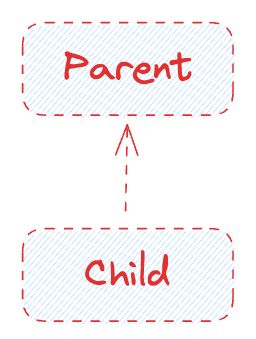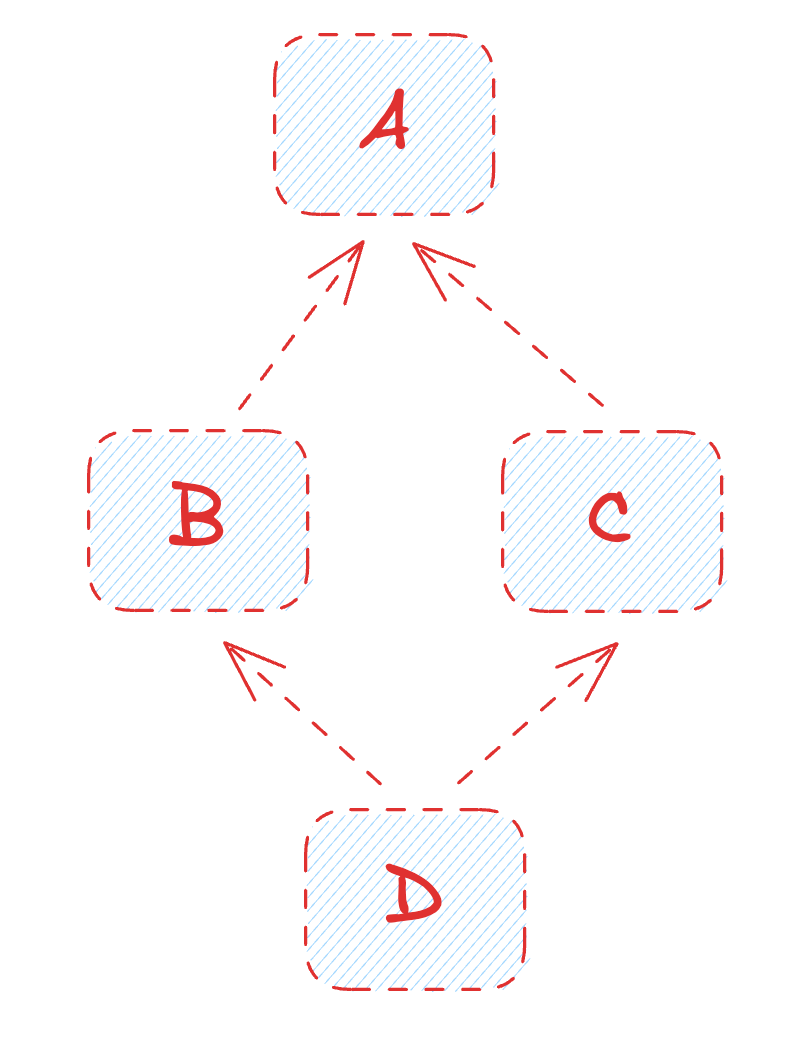# Python - super() 函式與 MRO 詳解

Posted on  Oct 6, 2023  in  Python 程式設計 - 中階  by  Amo Chen  ‐ 6 min read

Python 的物件導向程式設計(OOP)有 2 個一定要懂的東西：

1. super() 函式
2. MRO(Method Resolution Order) / 方法解析順序

`super() + MRO` = 超級瑪利歐？（誤

``````class Parent(object):
NAME = 'Parent'
def __str__(self):
return self.NAME

class Child(Parent):
NAME = 'Child'
def __str__(self):
return super().__str__()

c = Child()
print(c)
``````

• Python 3

### super() 函式介紹 #

``````class Parent(object):
def get_inheritance(self):
return 'Parent'
``````

``````class Child(Parent):
def get_inheritance(self):
return 'Parent <- Child'
````````````class Child(Parent):
def get_inheritance(self):
parent = super().get_inheritance()
return ' <- '.join([parent, 'Child'])

c = Child()
print(c.get_inheritance())
``````

``````Parent <- Child
``````

``````class Parent(object):
def get_inheritance(self):
return 'Parent'

class Child(Parent):
def get_inheritance(self):
parent = super().get_inheritance()
return ' <- '.join([parent, 'Child'])

class GrandChild(Child):
def get_inheritance(self):
parent = super().get_inheritance()
return ' <- '.join([parent, 'GrandChild'])

c = GrandChild()
print(c.get_inheritance())
``````

``````Parent <- Child <- GrandChild
``````#### super() 函式的應用 #

``````class Icon:
def __str__(self):
return '<i class="fa-solid fa-check"></i>'

class IconWithText(Icon):
def __init__(self, text):
self.text = text
def __str__(self):
return super().__str__() + f'<span>{self.text}</span>'

print(IconWithText('Hello'))
``````

``````<i class="fa-solid fa-check"></i><span>Hello</span>
``````

#### super() 函式的誤區 #

``````class Parent(object):
NAME = 'Parent'
def __str__(self):
return self.NAME

class Child(Parent):
NAME = 'Child'
def __str__(self):
return super().__str__()

c = Child()
print(c)     # Child
``````

### MRO(Method Resolution Order) / 方法解析順序 #

``````class A:
def run(self):
print('run')

class B(A):
def __init__(self):
self.run()

B()
``````

``````class A(object):
def method(self):
return 'Class A'

class B(A):
def method(self):
return 'Class B'

class C(A):
def method(self):
return 'Class C'

class D(B, C):
def method(self):
m = super().method()
print(m)
return 'Class D'

d = D()
d.method()
``````

``````Class B
````````````super(D, self).method()
``````

1. `<類別名稱>.__mro__`
2. `<類別名稱>.mro()`

``````>>> print(D.__mro__)
(<class '__main__.D'>, <class '__main__.B'>, <class '__main__.C'>, <class '__main__.A'>, <class 'object'>)
``````

``````>>> print(D.mro())
[<class '__main__.D'>, <class '__main__.B'>, <class '__main__.C'>, <class '__main__.A'>, <class 'object'>]
``````

p.s. `object` 類別是 Python 所有類別之母，所以最後都是 `object`

`super()` 有了第 2 個參數，就知道 MRO 是什麼以及怎麼找方法。

``````class A(object):
def method(self):
return 'Class A'

class B(A): pass

class C(A):
def method(self):
return 'Class C'

class D(B, C):
def method(self):
m = super().method()
print(m)
return 'Class D'

d = D()
d.method()  # result: Class C
``````

``````class A(object):
def method(self):
return 'Class A'

class B(A): pass

class C(A):
def method(self):
return 'Class C'

class D(B, C):
def method(self):
m = super(C, self).method()
print(m)
return 'Class D'

d = D()
d.method()  # Class A
``````

### 多重繼承與 MRO 的實務應用 #

``````class FlyMixin(object):
def fly(self):
print('Fly!')

class DiveMixin(object):
def dive(self):
print('Dive!')

class SuperSubmarine(FlyMixin, DiveMixin): pass

s = SuperSubmarine()
s.fly()
s.dive()
``````

``````Fly!
Dive!
``````

### 同場加映——先呼叫 `super().__init__()` 還是後呼叫 `super().__init__()` ？ #

``````
class A:
def __init__(self):
self.name = 'a'

class B(A):
def __init__(self):
super().__init__()
self.name = 'b'

print(B().name)  # b
``````

``````class A:
def __init__(self):
self.name = 'a'

class B(A):
def __init__(self):
self.name = 'b'
super().__init__()

print(B().name)
``````

### 總結 #

`super()` 函式的運用是邁向高手的重要關卡，在此之前正確理解 MRO 更是絕對要做的基本功，結合 2 者，將可以在 Python OOP 世界裡更加自在。

Happy Coding!

Built-in Functions - super()

Python’s super() considered super!

Django - Class-based views mixins